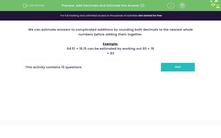# Use Rounding to Estimate Addition of Decimals

In this worksheet, students will estimate additions by rounding both decimals to the nearest whole numbers before adding them.Key stage:  KS 2

Curriculum topic:   Number: Fractions, Decimals and Percentages

Curriculum subtopic:   Solve Rounding Problems

Popular topics:   Rounding worksheets

Difficulty level:#### Worksheet Overview

We can estimate answers to complicated additions by rounding both decimals to the nearest whole number before adding them together.

How to round decimals to whole numbers

Look at the number directly to the right of the decimal point, that is the number in the tenths position.

If that number is less than 5, we round down so that we simply remove the numbers after the decimal point.

For example: 32.46 rounds down to 32 because the number in the tenths place is a 4.

159.08 rounds down to 159 because there is a zero in the tenths place.

If the number in the tenths place is 5 or more, we round up.

For example: 72.6 rounds up to 73 because the number in the tenths place is a 6.

197.57 rounds up to 198 because the number in the tenths place is a 5.

Having seen how to round decimals to the nearest whole number, let's look at how to use this to estimate addition of decimals.

Example:

64.51 + 18.15 can be estimated by rounding both decimals to the nearest whole number first: 64.51 rounds up to 65 and 18.15 rounds down to 18.

65 + 18 = 83

Let's have a go at some questions now.

### What is EdPlace?

We're your National Curriculum aligned online education content provider helping each child succeed in English, maths and science from year 1 to GCSE. With an EdPlace account you’ll be able to track and measure progress, helping each child achieve their best. We build confidence and attainment by personalising each child’s learning at a level that suits them.

Get started